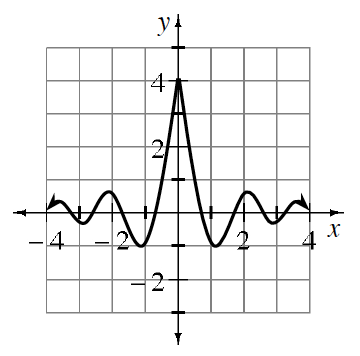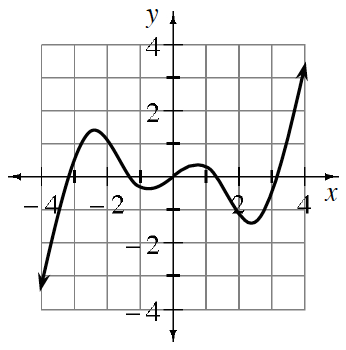### Home > APCALC > Chapter 1 > Lesson 1.1.1 > Problem1-10

1-10.

For each function sketched below, sketch $y=f(-x)$ and compare it with the original graph. Then describe its symmetry.

a.What does $f(−x)$ mean?
Compare $f(1)$ and $f(−1)$
Compare $f(2)$ and $f(−2)$
keep going... $f(3)$ and $f(−3)$

The sketch of $f(−x)$ should be identical to the given graph $f(x)$.

b.On this graph, $f(−x)=−f(x)$.

Plot all negative $y$-values in the positive region, and plot all positive $y$-values in the negative region.

c. EVEN AND ODD FUNCTIONS—INFORMALLY

A function that is symmetric with respect to the y-axis, like the one in part (a) above, is called an even function. A function that is symmetric with respect to the origin, like the one in part (b), is called an odd function.

Sketch examples of even and odd functions. Include how you can test whether a function is even or odd. Then list some famous even/odd functions that you have studied in a previous course, and the symmetries associated with even and odd functions.

Even functions are symmetrical ACROSS the $y$-axis, they have reflective symmetry. Odd functions are symmetrical ABOUT the origin, they have $180º$ rotational symmetry.

Graph (a) is even. Graph (b) is odd.

Famous even functions include: $y=x^2$, $y = x^4$, $y = \cos x$, and all vertical translations and stretches of the graphs above.

Famous odd functions include: $y = x$, $y = x^3$, $y = \sin x$, and all stretches of the graphs above.